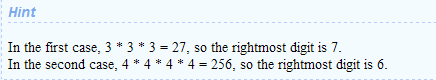# HDOJ 1197 Specialized Four-Digit Numbers

+关注继续查看

Problem Description
Find and list all four-digit numbers in decimal notation that have the property that the sum of its four digits equals the sum of its digits when represented in hexadecimal (base 16) notation and also equals the sum of its digits when represented in duodecimal (base 12) notation.

For example, the number 2991 has the sum of (decimal) digits 2+9+9+1 = 21. Since 2991 = 1*1728 + 8*144 + 9*12 + 3, its duodecimal representation is 1893(12), and these digits also sum up to 21. But in hexadecimal 2991 is BAF16, and 11+10+15 = 36, so 2991 should be rejected by your program.

The next number (2992), however, has digits that sum to 22 in all three representations (including BB016), so 2992 should be on the listed output. (We don’t want decimal numbers with fewer than four digits - excluding leading zeroes - so that 2992 is the first correct answer.)

Input
There is no input for this problem.

Output
Your output is to be 2992 and all larger four-digit numbers that satisfy the requirements (in strictly increasing order), each on a separate line with no leading or trailing blanks, ending with a new-line character. There are to be no blank lines in the output. The first few lines of the output are shown below.

Sample Input
There is no input for this problem.

Sample Output
2992
2993
2994
2995
2996
2997
2998
2999

（十进制的数范围[2992-9999]）

public class Main{
public static void main(String[] args) {
for(int i=2992;i<=9999;i++){
int a = i/1000+(i/100)%10+(i/10)%10+i%10;
String str = Integer.toString(i, 12);
//System.out.println(str);
int b = 0;
for(int j=0;j<str.length();j++){
switch(str.charAt(j)){
case 'a':b=b+10;break;
case 'b':b=b+11;break;
default:b=b+(new Integer(str.charAt(j)+""));
}
}
if(a!=b){
continue;
}
int c=0;
str = Integer.toString(i, 16);
//System.out.println(str);
for(int j=0;j<str.length();j++){
switch(str.charAt(j)){
case 'a':c=c+10;break;
case 'b':c=c+11;break;
case 'c':c=c+12;break;
case 'd':c=c+13;break;
case 'e':c=c+14;break;
case 'f':c=c+15;break;
default:c=c+(new Integer(str.charAt(j)+""));
}
}
if(a!=c){
continue;
}
System.out.println(i);
}

}

}HDOJ 1197 Specialized Four-Digit Numbers
HDOJ 1197 Specialized Four-Digit Numbers
0 0HDOJ 1061 Rightmost Digit（循环问题）
HDOJ 1061 Rightmost Digit（循环问题）
0 0HDOJ 2058 The sum problem
HDOJ 2058 The sum problem
0 0HDOJ 1001Sum Problem
HDOJ 1001Sum Problem
0 0HDOJ(HDU) 1720 A+B Coming(进制)
HDOJ(HDU) 1720 A+B Coming(进制)
0 0HDOJ 2029 Palindromes _easy version(回文串)
HDOJ 2029 Palindromes _easy version(回文串)
0 0HDOJ 2035 人见人爱A^B
HDOJ 2035 人见人爱A^B
0 0zoj 2406 Specialized Four-Digit Numbers
804 0HDOJ-1001 Sum Problem
Problem Description Hey, welcome to HDOJ(Hangzhou Dianzi University Online Judge).
910 0[LeetCode]233.Number of Digit One
1801 0

GitHub: https://github.com/chenhaoxiang